Galvanic cells (2021 VCE ) 1) Consider the following characteristics of electrolytic cells and galvanic cells.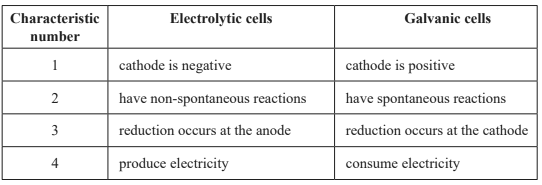Which of the following combinations of characteristics of electrolytic cells and galvanic cells are correct? A. only 1 and 2 B. only 2 and 3 C. only 3 and 4 D. only 1, 2 and 4 Solution2) A student hypothesised that polishing the zinc, Zn, electrode in an Fe–Zn galvanic cell would increase the current produced by the cell. What would be the most valid method of testing this hypothesis? A. researching the scientific literature to determine how polishing changes the structure of Zn B. measuring the conductivity of a Zn electrode after polishing it C. measuring the change in mass per unit time of the Fe electrode in the same Fe–Zn galvanic cell before and after the Zn electrode was polished D. measuring the current produced by two different Fe–Zn galvanic cells, one using a polished Zn electrode and the other using an unpolished Zn electrode Solution3) Different metal ion (aq)/metal (s) half-cells are combined with an In3+(aq)/In(s) half-cell to create a galvanic cell at SLC, as shown in the diagram below. The equation for the In3+(aq)/In(s) half-cell is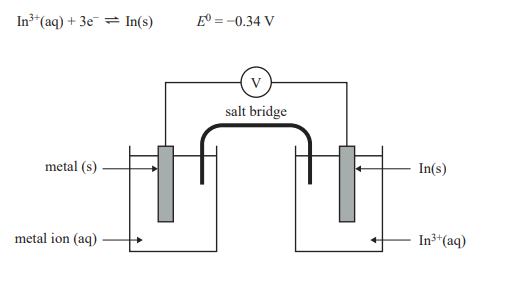Which of the following shows the half-cells in decreasing order of voltage produced when combined with the In3+(aq)/In(s) half-cell and In(s) is the negative electrode? A. Mn2+(aq)/Mn(s), Al3+(aq) / Al(s), Mg2+(aq)/Mg(s) B. Mg2+(aq)/Mg(s), Al3+(aq) / Al(s), Mn2+(aq)/Mn(s) C. Cu2+(aq)/Cu(s), Pb2+(aq) / Pb(s), Ni2+(aq)/Ni(s) D. Ni2+(aq)/Ni(s), Pb2+(aq) / Pb(s), Cu2+(aq)/Cu(s) Solution4) The following diagram shows two connected electrochemical cells.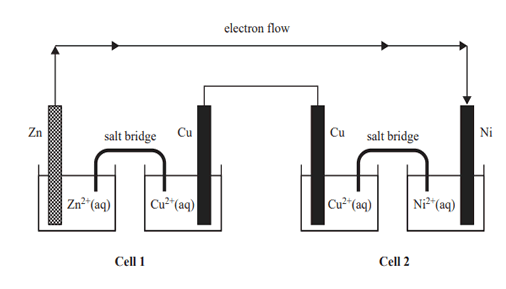Which of the following gives the energy transformations that occur in Cell 1 and in Cell 2?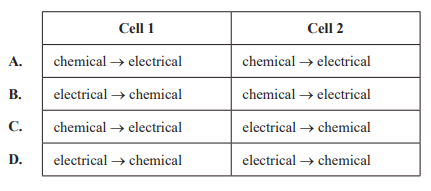Solution5) Research scientists are developing a rechargeable magnesium–sodium, Mg–Na, hybrid cell for use in portable devices. The Mg–Na hybrid cell uses magnesium metal and sodium ion electrodes and a hybrid organic/salt electrolyte, X. A simplified diagram of the rechargeable Mg–Na hybrid cell is shown below.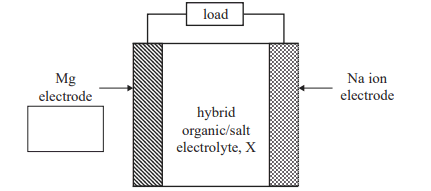a. The equation for the overall reaction during recharge is 2NaX + Mg2+ → Mg + 2Na+ + 2X i. Identify the polarity of the Mg electrode when the cell is discharging by placing a positive (+) or a negative (−) sign in the box provided in the diagram above. Solution ii. Write the half-cell equation of the reaction that occurs at the Mg electrode when the cell is discharging. Solution b. A pacemaker is a small electronic device that is implanted in the body to regulate a person’s heart rate. If the Mg–Na hybrid cell were to be used to power pacemakers, what would be two potential safety hazards of having this cell in the body? Solution c. One source of Mg is magnesium chloride, MgCl2, which can be obtained from seawater. Explain how Mg can be produced from MgCl2 in an electrolytic cell. Solution6) Rechargeable batteries A. use reversible reactions. B. operate as galvanic cells during recharge. C. require a continuous flow of reactants to operate. D. have fewer side reactions as temperature increases. Solution